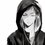# Is my solution correct?

Prove that :

$1 + \frac{1}{2} + \frac{1}{3} + .... + \frac{1}{n} \notin \mathbb{Z} (\forall n \in \mathbb{Z})$

Prove :

Assume $1 + \frac{1}{2} + \frac{1}{3} + .... + \frac{1}{n} \notin \mathbb{Z}$

So that : $1 + \frac{1}{2} + \frac{1}{3} + .... + \frac{1}{n} = \frac{a}{b} ; (a,b) = 1$

We must demonstrate : $1 + \frac{1}{2} + \frac{1}{3} + .... + \frac{1}{n+1} \notin \mathbb{Z}$

Pretend that : $1 + \frac{1}{2} + \frac{1}{3} + .... + \frac{1}{n+1} \in \mathbb{Z}$

$\Rightarrow \frac{a}{b} + \frac{1}{n + 1} \in \mathbb{Z}$ $\Rightarrow \frac{a(n + 1) + b}{b(n + 1)} \in \mathbb{Z}$ $\Rightarrow a(n + 1) + b \vdots b(n + 1)$

By (a,b) = 1 then $n + 1 \vdots b$ and $b \vdots n + 1$ so $n+1 = b$

But :

$1 + \frac{1}{2} + \frac{1}{3} + .... + \frac{1}{n} = \frac{a}{n+1} = \frac{c}{n!}$ Thus, $n! \vdots n+1 ;(absurdity)$

Therefore : $1 + \frac{1}{2} + \frac{1}{3} + .... + \frac{1}{n} \notin \mathbb{Z} (\forall n \in \mathbb{Z})$Note by Rony Phong
4 years, 11 months ago

This discussion board is a place to discuss our Daily Challenges and the math and science related to those challenges. Explanations are more than just a solution — they should explain the steps and thinking strategies that you used to obtain the solution. Comments should further the discussion of math and science.

When posting on Brilliant:

• Use the emojis to react to an explanation, whether you're congratulating a job well done , or just really confused .
• Ask specific questions about the challenge or the steps in somebody's explanation. Well-posed questions can add a lot to the discussion, but posting "I don't understand!" doesn't help anyone.
• Try to contribute something new to the discussion, whether it is an extension, generalization or other idea related to the challenge.

MarkdownAppears as
*italics* or _italics_ italics
**bold** or __bold__ bold
- bulleted- list
• bulleted
• list
1. numbered2. list
1. numbered
2. list
Note: you must add a full line of space before and after lists for them to show up correctly
paragraph 1paragraph 2

paragraph 1

paragraph 2

[example link](https://brilliant.org)example link
> This is a quote
This is a quote
    # I indented these lines
# 4 spaces, and now they show
# up as a code block.

print "hello world"
# I indented these lines
# 4 spaces, and now they show
# up as a code block.

print "hello world"
MathAppears as
Remember to wrap math in $$ ... $$ or $ ... $ to ensure proper formatting.
2 \times 3 $2 \times 3$
2^{34} $2^{34}$
a_{i-1} $a_{i-1}$
\frac{2}{3} $\frac{2}{3}$
\sqrt{2} $\sqrt{2}$
\sum_{i=1}^3 $\sum_{i=1}^3$
\sin \theta $\sin \theta$
\boxed{123} $\boxed{123}$

Sort by:

A simpler approach is to apply Bertrand's Postulate.

- 4 years, 11 months ago

That is an overkill for this problem. There is a much simpler approach.

Staff - 4 years, 11 months ago

Yes. It involves the highest power of 2.

- 4 years, 11 months ago

Good attempt. However, there is an error in the last line. So what if we have $\frac{a}{ n+1} = \frac{c}{n!}$? Why does that mean that $n! \mid n+1$? For example, $\frac{2}{4} = \frac{ 3}{6}$, and 4 and 6 do not divide each other.

If instead you want to argue that $n + 1 \not \mid n!$, then consider $n = 5$.

Staff - 4 years, 11 months ago

Thanks sir, but am I in the right track to moving on or i should change my methods ? Thanks in advance !

- 4 years, 11 months ago

You are most likely on the wrong track, in that I believe there is no solution arising from your current approach.

See Pi Han's comment stream for suggestions on how to solve this problem.

Staff - 4 years, 11 months ago